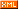# 简单，可复制

 :: 首页 :: 博问 :: 闪存 :: 新随笔 :: 联系 :: 订阅:: 管理 ::# 前言

原文翻译自http://www.cplusplus.com/doc/tutorial/typecasting/,觉得这篇文章讲C++类型转换简单明了，所以特别翻译了下。

在C++中，将一个已知的类型转换为另一个类型，我们称呼为类型转换,本文会介绍C++的各种类型转换。

# 隐式转换

short a=2000;int b;b=a;

class A {};class B { public: B (A a) {} };A a;B b=a;

# 显式转换

C++是一个强类型的语言。许多转换，需要显式转换,例如

short a=2000;int b;b = (int) a;    // c-like cast notationb = int (a);    // functional notation

// class type-casting#include <iostream>using namespace std;class CDummy {    float i,j;};class CAddition {    int x,y;  public:    CAddition (int a, int b) { x=a; y=b; }    int result() { return x+y;}};int main () {  CDummy d;  CAddition * padd;  padd = (CAddition*) &d;  cout << padd->result();  return 0;}

# C++标准转换运算符

dynamic_cast <new_type> (expression)reinterpret_cast <new_type> (expression)static_cast <new_type> (expression)const_cast <new_type> (expression)

# dynamic_cast

dynamic_cast只能用于指针和引用的对象其目的是确保类型转换结果是一个有效的完成所请求的类对象,所以当我们从一个类转换到这个类的基类，dynamic_cast总是可以成功

class CBase { };class CDerived: public CBase { };CBase b; CBase* pb;CDerived d; CDerived* pd;pb = dynamic_cast<CBase*>(&d);     // ok: derived-to-basepd = dynamic_cast<CDerived*>(&b);  // wrong: base-to-derived

dynamic_cast 可以转换NULL指针为不相关的类,也可以任何类型的指针为void指针。

dynamic_cast的需要运行时类型信息（RTTI）保持动态类型跟踪一些编译器支持此功能默认情况下是禁用选项必须启用运行时类型检查使用dynamic_cast的正常工作

# static_cast

static_cast可以执行相关的类指针之间转换不仅从派生类到基类的转换，也可以做到基类到派生类的转换。static_cast没有在运行时进行安全检查，因此，它是程序员，以确保转换是安全的，但是dynamic_cast的类型安全检查开销,static_cast是可以避免的。

class CBase {};class CDerived: public CBase {};CBase * a = new CBase;CDerived * b = static_cast<CDerived*>(a);

static_cast也可以用来执行任何其他非指针的转换例如像基本类型之间标准转换也可以隐式执行

double d=3.14159265;int i = static_cast<int>(d);

# reinterpret_cast

reinterpret_cast转换成任何其他指针类型甚至无关的类任何指针类型操作的结果是一个简单一个指针到其他二进制拷贝所有指针转换是允许的：不管是指针指向的内容还是指针本身的类型。

class A {};class B {};A * a = new A;B * b = reinterpret_cast<B*>(a)

# const_cast

const_cast用于操纵对象的常量性设置删除例如，一个函数要求一个非const参数,而程序需要传递一个const参数。

#include <iostream>using namespace std;void print (char * str){  cout << str << endl;}int main () {  const char * c = "sample text";  print ( const_cast<char *> (c) );  return 0;}

# typeid

typeid的允许检查表达式的类型
typeid (expression)

// typeid#include <iostream>#include <typeinfo>using namespace std;int main () {  int * a,b;  a=0; b=0;  if (typeid(a) != typeid(b))  {    cout << "a and b are of different types:\n";    cout << "a is: " << typeid(a).name() << '\n';    cout << "b is: " << typeid(b).name() << '\n';  }  return 0;}

typeid应用使用RTTI来跟踪动态对象类型,那么typeid的应用于表达式，其类型是一个多态，其结果是派生的最完整的对象类型

// typeid, polymorphic class#include <iostream>#include <typeinfo>#include <exception>using namespace std;class CBase { virtual void f(){} };class CDerived : public CBase {};int main () {  try {    CBase* a = new CBase;    CBase* b = new CDerived;    cout << "a is: " << typeid(a).name() << '\n';    cout << "b is: " << typeid(b).name() << '\n';    cout << "*a is: " << typeid(*a).name() << '\n';    cout << "*b is: " << typeid(*b).name() << '\n';  } catch (exception& e) { cout << "Exception: " << e.what() << endl; }  return 0;}

posted on 2012-01-04 10:20  ggjucheng  阅读(3328)  评论(0编辑  收藏  举报